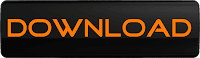• Instagram

Endothermic and Exothermic Reaction

Endothermic and Exothermic reaction

You will find those words if you have studied about chemistry. The endothermic and exothermic reaction is very important if we talk about the delta of temperature of a reaction.
These are the simplest definition about endothermic and exothermic reaction.
·         Endothermic reaction.
Endo mean inner, thermic mean temperature, and reaction mean reaction. So, we can conclude that endothermic reaction is a chemical reaction that absorb heat from surrounding that make the temperature of the reaction raises. So, if we measure the surrounding temperature, the surrounding temperature will decrease. See the illustration.Illustration of endothermal reaction

Because absorb the heat from surrounding so the deltaH of reaction is always + (positive)
Example
CaCO3(s) →  CaO(s) + CO2(g) ; DH = +178.5 kJ (with deltaH)
CaCO3(s) →  CaO(s) + CO2(g) – 178.5 kJ (without deltaH)
The other example of endothermic reaction is
KNO3(s) + H2O(l) → KNO3(aq)

·         Exothermic reaction.
Same with endothermic reaction, Exo mean outer, thermic mean temperature, and reaction mean reaction. So, we can conclude that exothermic reaction is a chemical reaction that release heat to surrounding that make the temperature of the reaction falls. So, if we measure the surrounding temperature, it will raises. See the ilustration.Illustration of exothermal reaction

Because release the heat to surrounding so the deltaH of reaction is always - (negative)
Example
C(s) + O2(g)  →  CO2(g) ; DH = -393.5 kJ (with deltaH)
C(s) + O2(g)  →  CO2(g) + 393.5 kJ (without deltaH)
The other example of endothermic reaction is
C3H8(g) + 3O2(g) → 3CO2(g) + 4H2O(l)
2Mg(s) + O2(g) → 2MgO(s)
HCl(aq) + NaOH(aq) → NaCl(aq) + H2O(l)
H2SO4(aq) + 2KOH(aq) → K2SO4(aq) + 2H2O(l)Click that button to download endo_and_exo_reaction.doc

Note :
Last link checked on April 15, 2013
The file is protected by a password

0 komentar:

Hey, It's my pleasure to know what was in your mind after reading the article above. So, you can comment or give critics to my writing on this comment box below